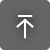Платные
ОС:
Язык:

## Описание

Add the length of multiple lines, multiple pair of points or multiple polylines and export the information to an Excel file or save the total distance to the clipboard.

The app will sum the length of consecutive points, of non-consecutive points of lines within a user-defined selection of polylines within a user-defined selection.Will sum all lines or polylines in model space that match the name of a user-defined layer

Customization of the output number with the help of a conversion factor that the user will define

This plugin has several summation options

• Add Consecutive Points: It will add the distance from one point to another point consecutively. For example from point P1 to p2 + p2 to p3 + p3 to p4
• Add non-consecutive points: It will add the distance between two points. For example the distance from point P1 to p2 + p3 to p4 + p5 + p6
• Add distance of lines with selection: Will sum the distance of only lines within a user-defined selection.
• Add distance of polylines with selection: Will sum the distance of only lines within a user-defined selection.
• Add all the LINES of a particular layer: will take the distance of all the lines in the model space and will add them only if they match the name of the layer that the user specifies
• Add all the POLYLINES of a particular layer: will take the distance of all the lines in the model space and will add them only if they match the name of the layer that the user specifies

Options

• Precision: defines the number of decimal places
• Factor: is a conversion factor to customize the output number, a number that will multiply the distance.

The user can add one of the following functions to complement the main option

• Exports the value of the total sum to the clipboard. To view this data, press Ctrl +V, if required in model space you will create a text with the saved length, in a word processing file you will paste the value
• Distance labelling

• It will add a text with the point to point distance or the lines/polylines, depending on the option chosen by the user. This function works well with the following options: text height. Prefix and an increment.
• The prefix: can be a letter For example "A-"
• The increment: will be a number, it is recommended that it be a whole number since a unit will be added for each line or pair of points.For example "5". As a result you will have, assuming that 3 lines are measured A-5 Distance, A-6 Distance, A-7 Distance and A-8 Distance.

• Export to Excel

If you opt for this option, it is recommended that you check the labelling option, otherwise in the Excel file you will only have the measurements, and you will not know to which line or pair of points they belong.

The output file will have two columns, one labelled with the word LINE and the other with the word

LINE| LENGTH

L-1.    1.23

L-2.    3.00

L-3.    2.01

L-4.    7.07

Total.  13.31

## Сведения об этой версии

Версия 1.0.0, 20.07.2022
First version.

## Рецензии клиентов

0 рецензия
Техническая поддержка